# Half Subtractor and Full Subtractor

Subtractor : Subtractor is the one which used to subtract two binary number(digit) and provides Difference and Borrow as a output.In digital electronics we have two types of subtractor.

1. Half Subtractor
2. Full Subtractor
Half Subtractor :Half Subtractor is used for subtracting one single bit binary digit from another single bit binary digit.The truth table of Half Subtractor is shown below.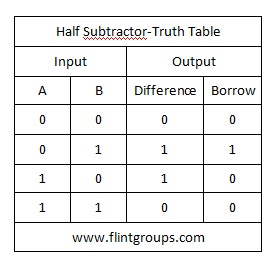Like Adders Here also we need to calculate the equation of  Difference and Borrow for more details please read What is meant by Arithmetic Circuits?

Difference = A'B+AB'=AB
Borrow=A'B

The logic Diagram of Half Subtractor is shown below.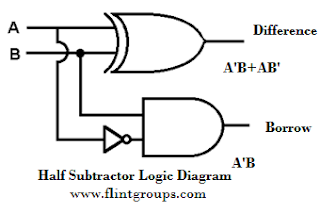Full Subtractor : A logic Circuit Which is used for Subtracting Three Single bit Binary digit is known as Full Subtractor.The Truth Table of Full Subtractor is Shown Below.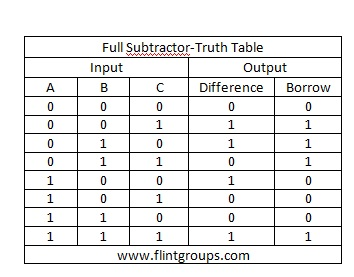From the Truth Table The Difference and Borrow will written as

Difference=A'B'C+A'BB'+AB'C'+ABC
Then We got
Difference=ABC
Borrow=A'B'C+A'BC'+A'BC+ABC
=A'B'C+A'BC'+A'BC+A'BC+A'BC+ABC    ---------->  A'BC=A'BC+A'BC+A'BC
=A'C(B'+B)+A'B(C'+C)+BC(A'+A)
Borrow=A'C+A'B+BC

The logic diagram of Full Subtractor is Shown below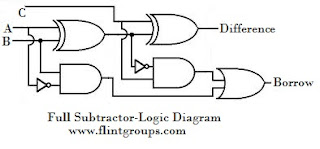1.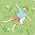This was very neat and simple to understand. Thank you!

2.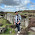3.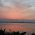It Just Awesome!!! :-)

4.It's very easy to understand

5.It's very easy to understand

6.i cannot understand the substraction part...how it is 0110 it should be 0010....as 12-10 is 2 which is 0010

7.i cannot understand the substraction part...how it is 0110 it should be 0010....as 12-10 is 2 which is 0010

1.Its not like u seen it in a different way.

0 - 0 difference is 0 borrow is also 0

0 - 1 1 is greater than 0 so we need a borrow to subtract 1 from 0 if u get the borrow then difference is 1 and borrow is 1.

1 - 0 difference is 1 and borrow is 0

1 - 1 difference is 0 and borrow is also 0 .

don't think as 0011 - 0101.

I think you are clear now.till you are not clear don't hesitate to contact me.

2.you need 4 full subtractors to perform the operations like 0011 - 0101

8.This comment has been removed by the author.

9.This comment has been removed by the author.

10.11.Simple And Neat Diagram Thnx Flint

12.Simple And Neat Diagram Thnx Flint

13.Thanks for giving very simple language to understand...

14.15.It not enough ..

1.16.NICE EXPLANATION....THANKS

17.Which digit is subtracting from another digit I.e A-B. Or B-A

18.B-C-A .isn't it?

19.20.It was awsome...

21.Neat , sharp. wonderful. Thanks a lot

22.When I try to solve the difference in boolean simplification, i am always getting a value of 1, how youve come up the result of A+B+C with a circle on the operator?

1.I was not able to understand what you are asking can you elaborate your query

2.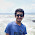remember that ~(A.B) is not (~A.~B)
it is (~A+~B)

23.Thank you this is very simple

24.That's a really nice and simple explanation. I have also a similar article on Half adder and full adder

25.Short and easy